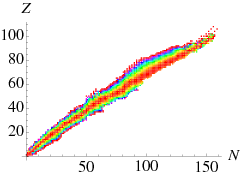$$\require{cancel}$$

# 4.5: Stability of Nuclei

•• Contributed by Niels Walet
• Professor (Physics) at University of Manchester

In Figure $$\PageIndex{1}$$ we have color coded the nuclei of a given mass $$A=N+Z$$ by their mass, red for those of lowest mass through to magenta for those of highest mass. We can see that typically the nuclei that are most stable for fixed $$A$$ have more neutrons than protons, more so for large $$A$$ increases than for low $$A$$. This is the “neutron excess”.Figure $$\PageIndex{1}$$: The valley of stability

# $$\beta$$ decay

If we look at the mass of nuclides with fixed nucleon number $$A$$ (i.e., roughly perpendicular cuts through the valley of stability in Figure $$\PageIndex{1}$$), we can see that the masses vary strongly,Figure $$\PageIndex{2}$$: The negative of binding energy per nucleon for nuclides with ﬁxed $$A$$: (left) $$A= 56$$ and (right) $$A= 150$$. The profile of binding energy across the valley of stability is roughly a parabola (e.g., Iron-56 is stable, while Vandium-56 is unstable to $$β^−$$ decay.

It is known that a free neutron is not a stable particle, it actually decays by emission of an electron and an antineutrino,

$n \rightarrow p + e^-+\bar{\nu}_e.$

The reason that this reaction can take place is that it is endothermic,

$m_n c^2 > m_p c^2 + m_e c^2.$

Here we assume that the neutrino has no mass.

The degree of allowance of such a reaction is usually expressed in a $$Q$$ value, the amount of energy released in such a reaction,

\begin{align} Q &=m_n c^2 - m_p c^2 - m_e c^2 \\[5pt] &=939.6-938.3-0.5=0.8\text{ MeV}. \end{align}

Generically it is found that two reaction may take place, depending on the balance of masses. Either a neutron “$$\beta$$ decays” as sketched above, or we have the inverse reaction

$p \rightarrow n + e^++{\nu}_e.$

For historical reason the electron or positron emitted in such a process is called a $$\beta$$ particle. Thus in $$\beta^-$$ decay of a nucleus, a nucleus of $$Z$$ protons and $$N$$ neutrons turns into one of $$Z+1$$ protons and $$N-1$$ neutrons (moving towards the right in Figure $$\PageIndex{2A}$$. In $$\beta^+$$ decay the nucleus moves to the left. Since in that figure I am using atomic masses, the $$Q$$ factor is

\begin{aligned} Q_{\beta^-}&= M(A,Z)c^2-M(A,Z+1)c^2,\nonumber\\ Q_{\beta^-}&= M(A,Z)c^2-M(A,Z-1)c^2-2m_e c^2.\end{aligned}

The double electron mass contribution in this last equation because the atom looses one electron, as well as emits a positron with has the same mass as the electron.

In similar ways we can study the fact whether reactions where a single nucleon (neutron or proton) is emitted, as well as those where more complicated objects, such as Helium nuclei ($$\alpha$$ particles) are emitted. I shall return to such processed later, but let us note the $$Q$$ values,

\begin{aligned} \text{neutron emission} && Q&=[M(A,Z)-M(A-1,Z)-m_n]c^2, \nonumber\\ \text{proton emission} && Q&=[M(A,Z)-M(A-1,Z-1)-M(1,1)]c^2, \nonumber\\ \text{\alpha emission} && Q&=[M(A,Z)-M(A-4,Z-2)-M(4,2)]c^2, \nonumber\\ \text{break up} && Q&=[M(A,Z)-M(A-A_1,Z-Z_1)-M(A_1,Z_1)]c^2.\end{aligned}